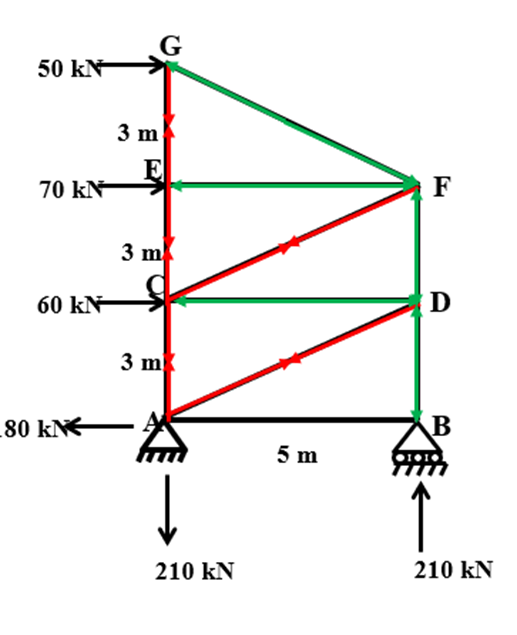### Analysis of Truss

Definitions

• Joint
Points are considered as joint. It is a connection of two or more members.

• Member
Lines are considered as members. It is an element between two joints.

In a truss, the joints are of pin type, where the end of the members can rotate freely. Moreover, individual truss members should not directly loaded transversely; loads should be applied at the joints of members

Assumptions in the Theory of Truss

• All members are straight and connected at the ends by pin-connection (hinged).

• The loads act only at the joints.

• Self-weights of the members are neglected.

• Cross-section of the members is uniform.

• The members of truss carry on axial forces either be in tension or in compression.

The analysis of the truss is by two methods:

1. Method of Joint, and

2. Method of section

Method of Joint

In this method, a free body diagram of each joint is drawn and equations of static equilibrium are used to solve for the unknown at that joint.

Steps of Analysis

1. Determine whether truss is perfect or not using the equation, m = 2j-3.

2. Determine support reactions using,

3. Consider any one joint which has only two unknowns from visual observations.

4. Draw Free Body Diagrams of that joint. Assume direction of the unknown forces in FBD from geometry.

5. Find angles of each member w.r.t horizontal or vertical axes.

6. Apply equilibrium equations at that joint  and find unknown forces.

7. Consider any new joint which has also two unknowns from visual observations of the truss.

8. Repeat Step 4 to 7 for all joints and find unknown forces in each members of truss till complete solution is arrived.

Example: (Analysis of Plane Truss by Method of Joint)
Determine member forces in all the members of the truss as shown in Figure: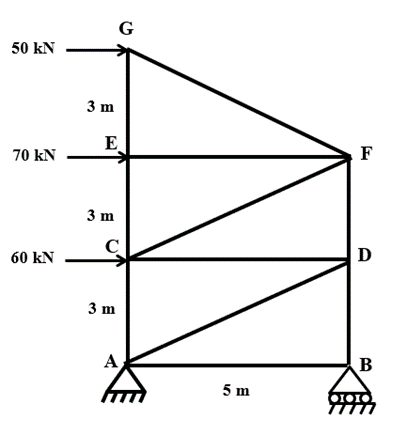Sign conventions:

Left side forces (→) are considered as +ve
Upward Forces (↑) are considered as +ve

Step-1: To determine support reactions:
At joint A (hinge) there are two unknown reaction (HA and VA) and At Joint B there is one unknown reaction (VB). Apply equilibrium conditions: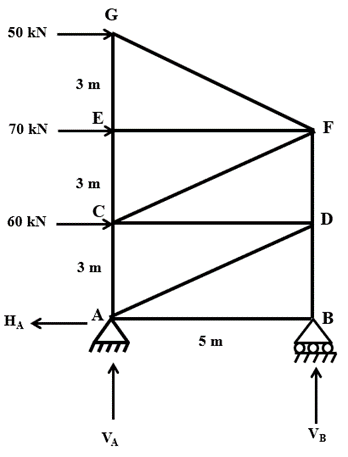∑H = 0

∑V = 0

∑M = 0, Taking moment @ A

Now consider each joint one by one. Draw a free body diagram and determine unknown member forces in it.
Joint G: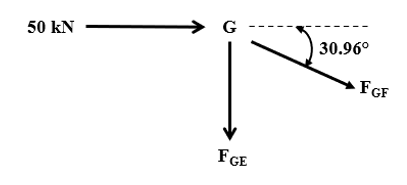Joint-E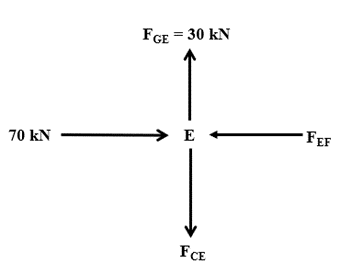Joint F: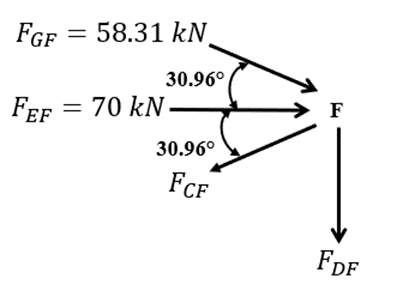Joint C: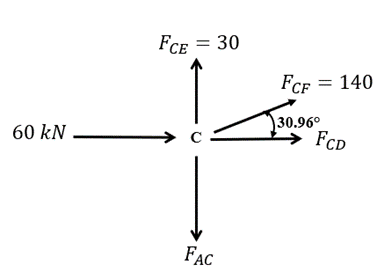Joint A: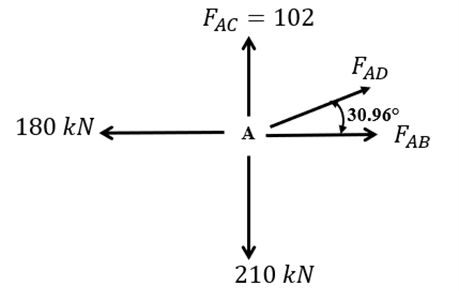Summary:

 SR.No Member Force (kN) Nature 1 AB 0 - 2 AC 102 Tension 3 AD 210 Tension 4 BD 210 Compression 5 CD 180 Compression 6 CE 30 Tension 7 CF 140 Tension 8 DF 102 Compression 9 EF 70 Compression 10 EG 30 Tension 11 FG 58.31 Compression